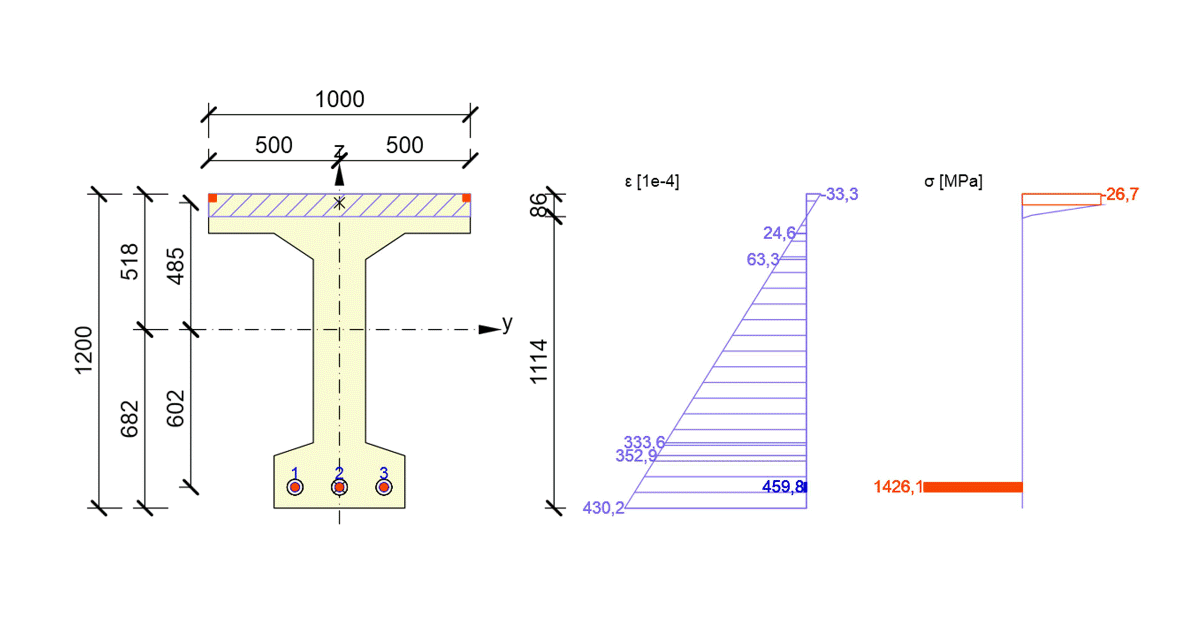### Choose language# Moment of resistance of prestressed section - comparison of hand calculations and IDEA StatiCa

$$In this article, a moment of resistance of an “I” section subjected to the combination of tensile axial force and bending moment due to the external load and prestressing (including secondary effects) is calculated. Two different methods are used to calculate the flexural resistance of a prestressed cross-section and then compared to each other. In spite of the fact that the methods used for the calculation are completely different, the results are almost identical. Attached Downloads I_section_assessment.pdf (PDF, 2.3 MB)$$